OUTPUT Statement
OUTPUT <OUT=SAS-data-set> < keyword=name ...keyword=name> </options> ;

The OUTPUT statement creates a new SAS data set containing statistics calculated for each observation. These can include the estimated linear predictor (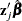) and its standard error, survival distribution estimates, residuals, and influence statistics. In addition, this data set includes the time variable, the explanatory variables listed in the MODEL statement, the censoring variable (if specified), and the BY, STRATA, FREQ, and ID variables (if specified).

For observations with missing values in the time variable or any explanatory variables, the output statistics are set to missing. However, for observations with missing values only in the censoring variable or the FREQ variable, survival estimates are still computed. Therefore, by adding observations with missing values in the FREQ variable or the censoring variable, you can compute the survivor function estimates for new observations or for settings of explanatory variables not present in the data without affecting the model fit.

No OUTPUT data set is created if the model contains a time-dependent variable defined by means of programming statements.

The following list explains specifications in the OUTPUT statement.

OUT=SAS-data-set

names the output data set. If you omit the OUT= option, the OUTPUT data set is created and given a default name by using the DATAn convention. See the section OUT= Output Data Set in the OUTPUT Statement for more information.

keyword=name
specifies the statistics included in the OUTPUT data set and names the new variables that contain the statistics. Specify a keyword for each desired statistic (see the following list of keywords), an equal sign, and either a variable or a list of variables to contain the statistic. The keywords that accept a list of variables are DFBETA, RESSCH, RESSCO, and WTRESSCH. For these keywords, you can specify as many names in name as the number of explanatory variables specified in the MODEL statement. If you specify k names and k is less than the total number of explanatory variables, only the changes for the first k parameter estimates are output. The keywords and the corresponding statistics are as follows:
ATRISK

specifies the number of subjects at risk at the observation time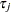(or at the right endpoint of the at-risk interval when a counting process MODEL specification is used).

DFBETA

specifies the approximate changes in the parameter estimates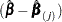when theth observation is omitted. These variables are a weighted transform of the score residual variables and are useful in assessing local influence and in computing robust variance estimates.

LD

specifies the approximate likelihood displacement when the observation is left out. This diagnostic can be used to assess the impact of each observation on the overall fit of the model.

LMAX

specifies the relative influence of observations on the overall fit of the model. This diagnostic is useful in assessing the sensitivity of the fit of the model to each observation.

LOGLOGS

specifies the log of the negative log of SURVIVAL.

LOGSURV

specifies the log of SURVIVAL.

RESDEV

specifies the deviance residual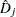. This is a transform of the martingale residual to achieve a more symmetric distribution.

RESMART

specifies the martingale residual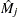. The residual at the observation timecan be interpreted as the difference over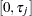in the observed number of events minus the expected number of events given by the model.

RESSCH

specifies the Schoenfeld residuals. These residuals are useful in assessing the proportional hazards assumption.

RESSCO

specifies the score residuals. These residuals are a decomposition of the first partial derivative of the log likelihood. They can be used to assess the leverage exerted by each subject in the parameter estimation. They are also useful in constructing robust sandwich variance estimators.

STDXBETA

specifies the standard error of the XBETA predictor,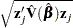.

SURVIVAL

specifies the survivor function estimate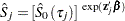, whereis the observation time.

WTRESSCH

specifies the weighted Schoenfeld residuals. These residuals are useful in investigating the nature of nonproportionality if the proportional hazard assumption does not hold.

XBETA

specifies the estimate of the linear predictor,.

The following options can appear in the OUTPUT statement after a slash (/) as follows:

ORDER=value

specifies the order of the observations in the OUTPUT data set. The following values are available:

DATA

requests that the output observations be sorted the same as the input data set.

SORTED

requests that the output observations be sorted by strata and descending order of the time variable within each stratum.

The default is ORDER=DATA.

METHOD=method
specifies the method used to compute the survivor function estimates. The following methods are as available:
CH
EMP

specifies that the empirical cumulative hazard function estimate of the survivor function be computed; that is, the survivor function is estimated by exponentiating the negative empirical cumulative hazard function.

PL

specifies that the product-limit estimate of the survivor function be computed.

The default is METHOD=BRESLOW.